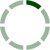### Quizzes/Exams for Package 2

Our core fundamental concepts in finance involve the basic financial modeling and valuation techniques that introduce model building best practices as well as getting used to working efficiently in Excel. After understanding the basic fundamental concepts, the most important building blocks of modeling are introduced as we begin to thoroughly analyze financial statements and their implications. We introduce the underpinnings of fundamental valuation (i.e. DCF analysis) and relative valuation (comps & multiples).

### Last 10 posts

No posts